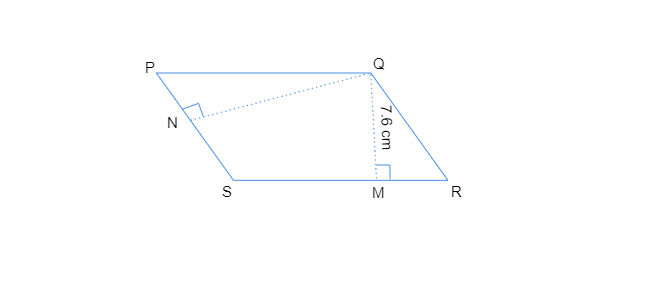# $PQRS$ is a parallelogram $(Fig 11.23)$. $QM$ is the height from $Q$ to $SR$ and $QN$ is the height from $Q$ to $PS$. If $SR = 12\ cm$ and $QM = 7.6\ cm$. Find:$(a)$ the area of the parallegram $PQRS$ $(b)$ $QN$

$(a)$. $SR = 12\ cm$ and $QM = 7.6\ cm$

Area of parallelogram $PQRS = Base\times Height$

$= SR\times QM$

$= 12\ cm\times 7.6\ cm$

$= 91.2 cm^2$

$(b)$. Base $= PS = 8\ cm$

Area of the parallelogram $= 91.2\ cm^2$       [calculated in part $(a)$]

Area of parallelogram $PQRS = Base\times Height$

$91.2\ cm^2 = 8 cm\times QN$

$QN = 91.2\ cm^2 / 8 cm$

$QN = 11.4\ cm$